# How to use the INDEX function in Google SheetsThe INDEX function in Google Sheets returns the value of a cell within an input range, relatively separated from the first cell by row and column offsets. This is similar to the index at the end of a book, which provides a quick way to locate specific content.

### Syntax

INDEX(reference, [row_offset], [column_offset])

• reference – the address of the range of cells within which the offset is evaluated from the very first cell (on the top left). Accordingly, the INDEX formula returns the value of the offset target cell.
• row_offset – the number of rows to offset from the starting cell.
• column_offset – the number of columns to offset from the starting cell.

### How to use the INDEX function in Google Sheets

Let’s take a look at some practical examples to understand how to use this function.

In the following examples I’ve used some fictitious data to show the INDEX function in use.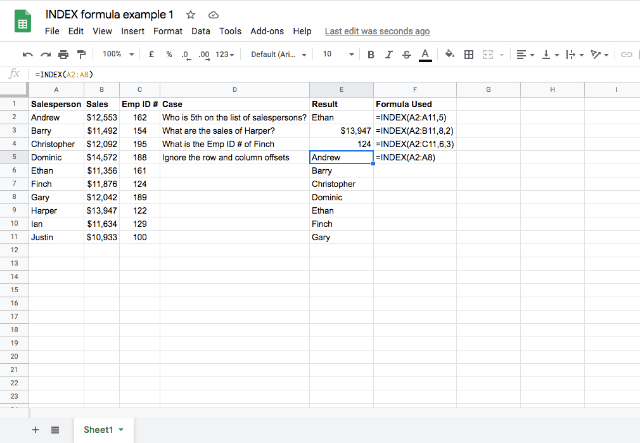In the above screenshot, all the cases except the last one are pretty straightforward.

Essentially, you give the formula a range of cells, then give it the coordinates of the cell in the range that you want it to return as the result. So in row 3, where the formula is =INDEX(A2:B11,8,2), I’m telling the formula that I want the cell in row 8, column 2 of the array, which is \$13,947.

In the last example in the screenshot (row 5) I did not specify any row or column offsets. As a result, the function returned the complete set of values from the input reference range. If anything, this could serve as an input for another array formula. For instance, you could use this to consolidate data from multiple sheets, or you could do something like =COUNTA(INDEX(A2:A8)), that would have returned the value 7.

Are there any cases where INDEX function might throw up an error? Of course, yes! When you try to point the function away from the confines of the input range, it does cough up an error, as shown in the screenshot below: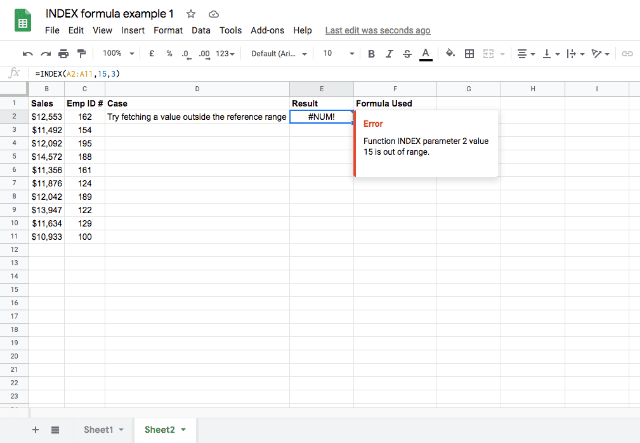### How to use INDEX function in combination with other formulas

Here I’ll take a look at some use cases that demonstrate how Google Sheets INDEX function can be used in combination with other functions and formulas.

#### Use Case #1: INDEX function and COUNTA function

Combining the COUNTA function with the INDEX function can be useful in situations where, for instance, you always want to perform a calculation using the last row of data in a list that is regularly being added to.

In the following screenshot, I have a spreadsheet that gets updated every week with the average temperature for that week.

If I always want to perform a calculation with the most recent week’s average temperature, I can use COUNTA and INDEX to always select the last entry, using the formula =INDEX((A:B), COUNTA(A:A),2)Here, the entire A and B columns are the range. The COUNTA(A:A) function counts the number of data points in column A and tells you how many there are, and the INDEX formula takes that number as the row that it will get its result from.

The 2 at the end tells the formula that it will get the result from the second column of the range (column B). So no matter how many weeks you add to the list, the “Most recent week” will always stay up-to-date!

### Use Case #2: combining MATCH and INDEX functions

Perhaps one of the most powerful uses of INDEX function in Google Sheets is when it is used along with MATCH, in order to look up values.

But there is already a VLOOKUP formula in Google Sheets for that purpose, right?

Take a look at the example below: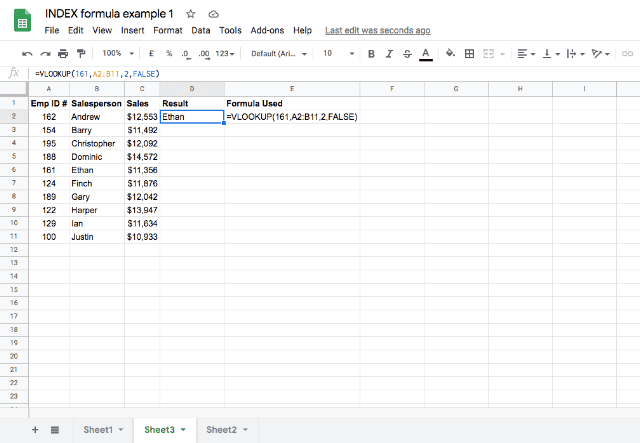The VLOOKUP formula in D2 looks up 161 in the Emp ID # column (as it’s the leftmost in the range A2:B11), and from the row where it finds the value 161, it fetches the value located in the second column (i.e. Column B), while assuming the data is not sorted.

So far, so good. But there are two critical problems with VLOOKUP in Google Sheets.

### Problems with VLOOKUP that can be solved using the INDEX and MATCH functions

Before going further with examples of how to use the INDEX and MATCH functions in combination, I will outline two critical issues with the VLOOKUP, in order to give you an idea of the limitations of this function and when to use INDEX and MATCH instead.

#### Problem #1: Static cell referencing

What happens when you insert a new column between the first and second columns? Let’s try that: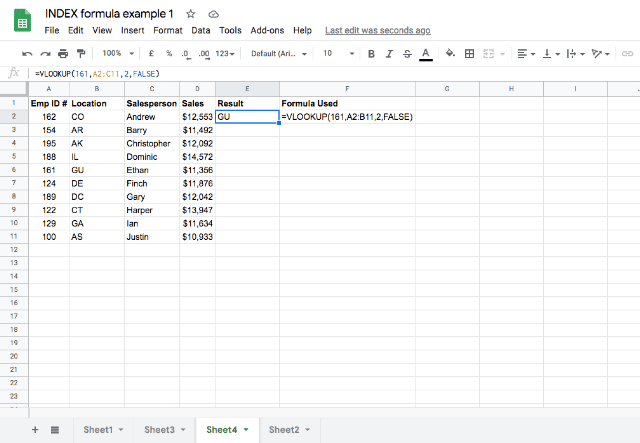You’ll notice the returned value is not “Ethan” anymore. This is because VLOOKUP is a semi-static formula.

Google Sheets updated the second parameter to reflect the new range, but it did not accordingly change the column index (third parameter) when a new column was added before the Salesperson column.

#### Problem #2: Lookup column is always the leftmost

Situations may arise where you might have to lookup values from a column (Emp ID #) that is not the leftmost, as shown below.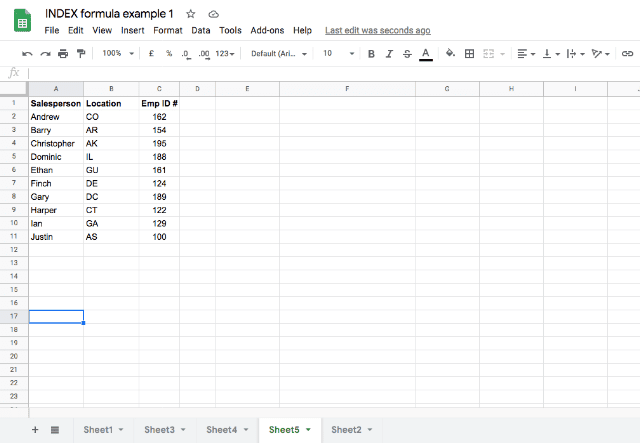In this case, moving the Emp ID # column to make it the leftmost would work. But that isn’t an ideal approach – there can be data layout or presentation specifications that do not allow you to re-arrange columns.

In that situation, what can you do? The MATCH and INDEX combination comes to the rescue. Here’s the syntax, followed by a few examples.

INDEX(reference, MATCH(search_key, range, search_type))

The key to this combination is that both the ranges selected for the INDEX and MATCH functions, respectively, need to be a single column.

You are essentially using a VLOOKUP, but specifying the column to look in, and the column to return the value from, in separate ranges within the MATCH and INDEX functions As you’ll see, this methodology helps you avoid the errors that can occur using VLOOKUP.

### MATCH function

I’ll give a brief summary of the MATCH function here for those who are not familiar with it. The MATCH function takes a given search_key, which is that value that you’re telling it to look for within a certain range. Unlike with the INDEX function, this needs to be one-dimensional (within just one column or one row).

The MATCH function gives you the location of the item you’ve told it to search for. For search_type you typically say 0, which tells the function it needs to be an exact match.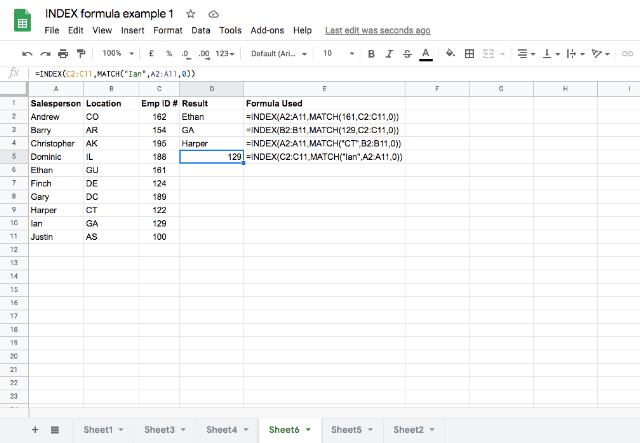In the above example, I’ve used the MATCH function to tell me which row contains Emp ID #161 within column C. The function returns a number that tells the INDEX function which row in column A to look for the Salesperson. The result is that the two functions combine to tell me the correct name (Ethan) for Emp ID #161. The formula I used to do this is =INDEX(A2:A11,MATCH(161,C2:C11,0)).

As you can see, unlike VLOOKUP, the combination works even if the lookup column is not the leftmost (first three examples in the above screenshot). Not surprisingly, it also works like VLOOKUP, when the lookup column is the leftmost.

Let’s also see whether the functions hold up when you introduce a new column in between.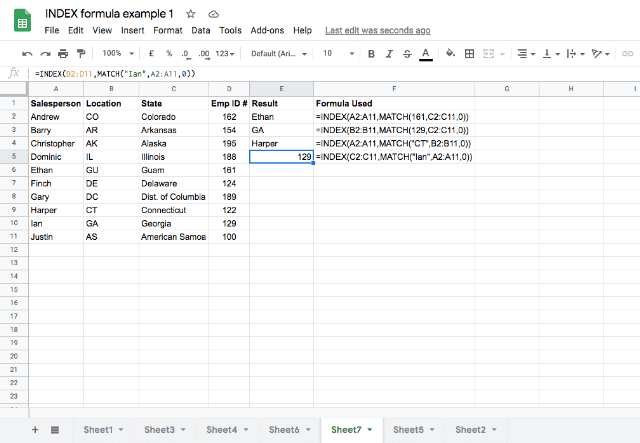Thankfully, they still work. As soon as I introduced a new column (State), Google Sheets updated the references automatically to accommodate this change.

As you’ve seen above, the MATCH and INDEX function combination is much more flexible and versatile than the already popular and powerful VLOOKUP function. But the combination of these functions can be even more powerful when you use two MATCH functions instead of just one.

### Use case #3: INDEX function with two MATCH functions

You’ve now seen how useful the INDEX and MATCH combination can be. But what if you have a bi-dimensional array from which you want to get a value? In this case, you can use the MATCH function twice within the INDEX function, as shown in the example below.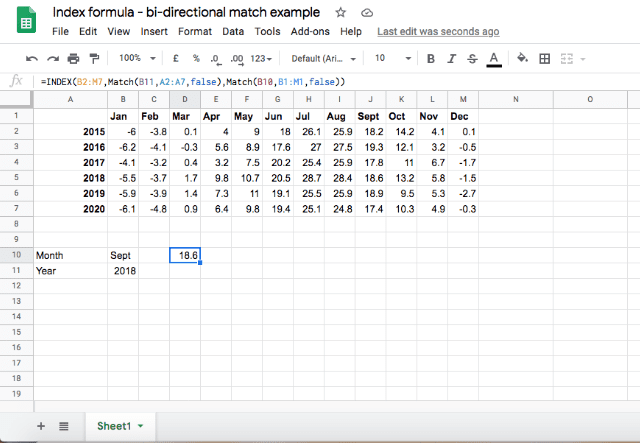In this example, you can see data on the average temperature in each month, for the years 2015-2020. If you want to pull the average temperature of a specific month of a specific year out of this array, you can use the INDEX and MATCH combination to accomplish this. The function used here is:

=INDEX(B2:M7, Match(B11, A2:A7, false), Match(B10, B1:M1, false))

As you can see, I am using the MATCH function to give me the location of the row for the year I am looking for (in this case it’s 2018, which is in the 4th row of data) as well as the column for the month I am looking for (September, in the 9th column of data). The INDEX function then takes these coordinates and returns the average temperature for September 2018.

By using the INDEX and MATCH functions, you can not only get a search function that is more versatile than VLOOKUP, but you can also search bi-dimensional arrays. Learn more about Google Sheets MATCH function here.

#### Did you like this post?

If you found this article useful, share it with your network via the social media buttons on the left!

Editor’s note: This is a revised version of a previous post that has been updated for accuracy and comprehensiveness.